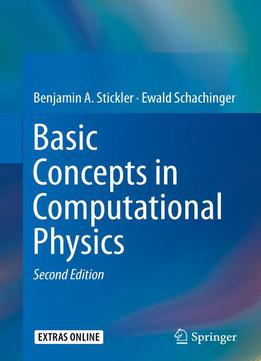# Basic Concepts In Computational Physics, Second Edition by Benjamin A. Stickler / 2016 / English / EPUB

This new edition is a concise introduction to the basic methods of computational physics. Readers will discover the benefits of numerical methods for solving complex mathematical problems and for the direct simulation of physical processes.

The book is divided into two main parts: Deterministic methods and stochastic methods in computational physics. Based on concrete problems, the first part discusses numerical differentiation and integration, as well as the treatment of ordinary differential equations. This is extended by a brief introduction to the numerics of partial differential equations. The second part deals with the generation of random numbers, summarizes the basics of stochastics, and subsequently introduces Monte-Carlo (MC) methods. Specific emphasis is on MARKOV chain MC algorithms. The final two chapters discuss data analysis and stochastic optimization. All this is again motivated and augmented by applications from physics. In addition, the book offers a number of appendices to provide the reader with information on topics not discussed in the main text.

Numerous problems with worked-out solutions, chapter introductions and summaries, together with a clear and application-oriented style support the reader. Ready to use C++ codes are provided online.

views: 774